# Multiply and divide decimals by 10, 100 and 1000

## Home learning focus

Learn how to multiply and divide decimals by 10, 100 and 1000.

This lesson includes:

• one video
• one interactive activity
• two worksheets

# Learn

Did you know that when you multiply and divide a decimal by 10, 100 and 1000, the place value of the digits either increases or decreases?

• When multiplying, the digits move to the left since the number gets bigger.
• When dividing, the digits move to the right since the number gets smaller.

It is important to remember that the decimal point does not move.

## Decimals in daily life

Multiplying and dividing decimals helps you in everyday life. It can help you to:

• convert pounds into pence and vice versa.
• convert measures such as kilograms to grams and millilitres to litres and vice versa when baking.
• convert length, for example if you needed to work out how much space a small wardrobe would take up in a room measured in metres.

You can use place value charts to help multiply and divide by 10, 100 and 1000. This enables you to see how the digits increase or decrease in value.

A trick to help you remember how many places the digits need to move is by looking at the zeros in 10, 100 and 1000.

For example, if you multiply or divide by 1000, you either move 3 places to the right or left.

• 1 zero = 1 place
• 2 zeros = 2 places
• 3 zeros = 3 places

## Multiplying and dividing by 10

This video will help you to understand place value and how it can be used to multiply and divide decimals by 10. Why not make some notes as you watch the video to help you to remember later?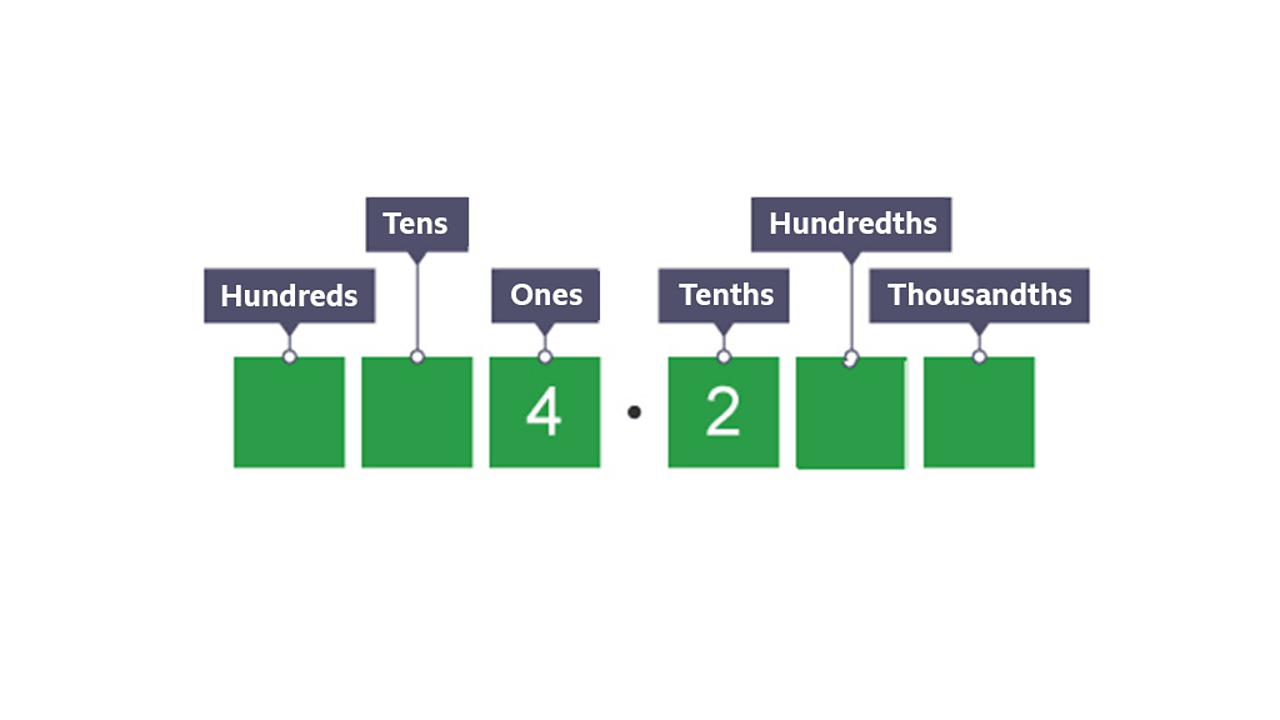Multiplying by 10What is 4.2 x 10?1 of 4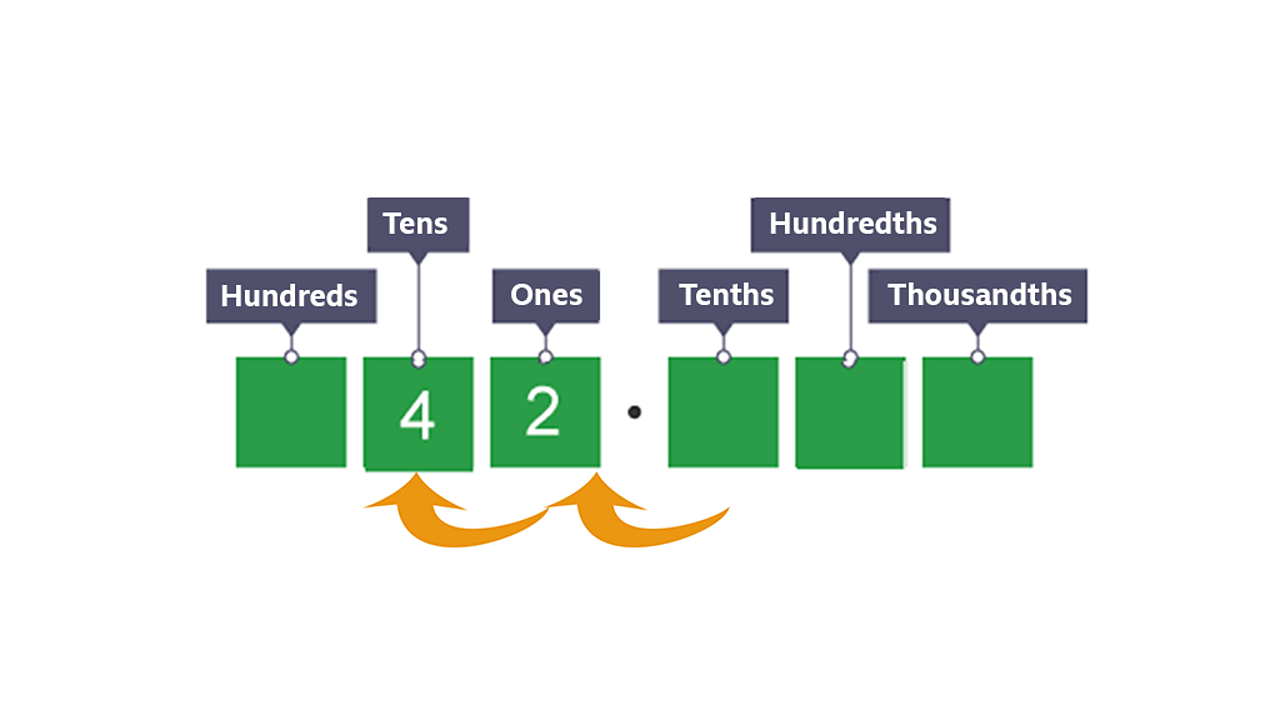To multiply by 10, you move the digits one place value to the left. So 4.2 x 10 = 42. We don’t have any numbers after the decimal point now so we don’t have to include it!2 of 4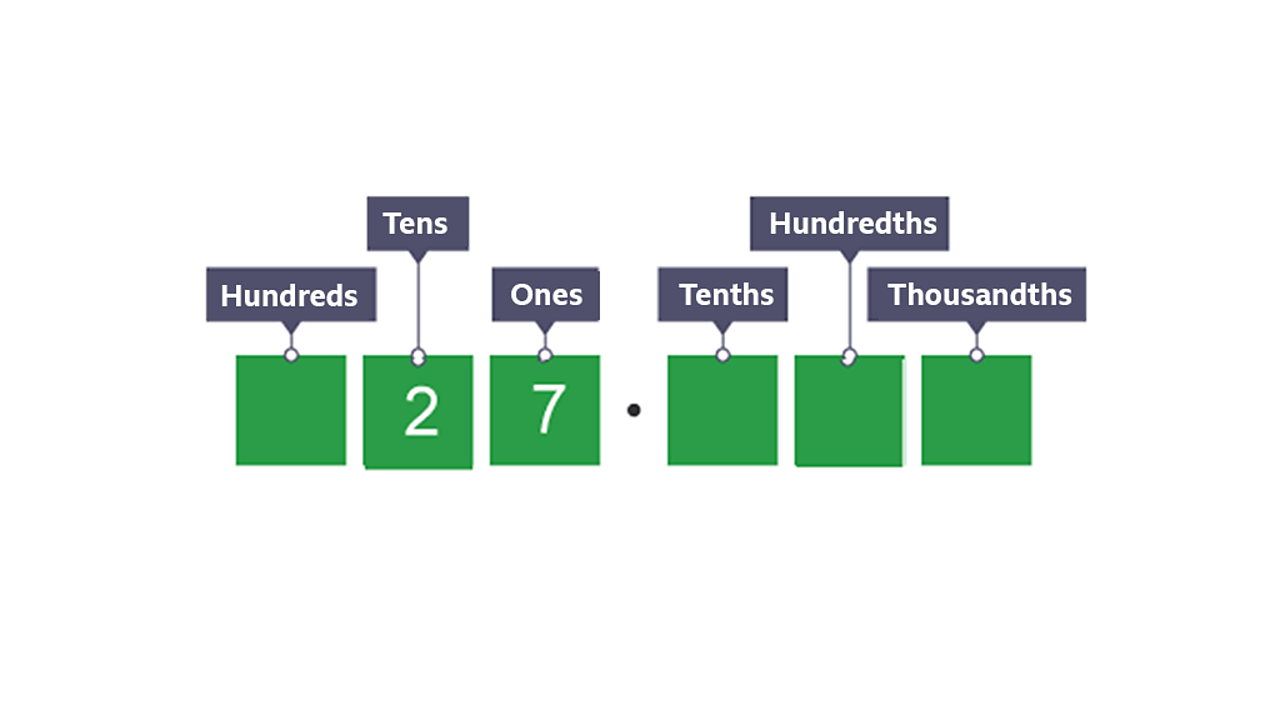Dividing by 10What is 27 divided by 10?3 of 4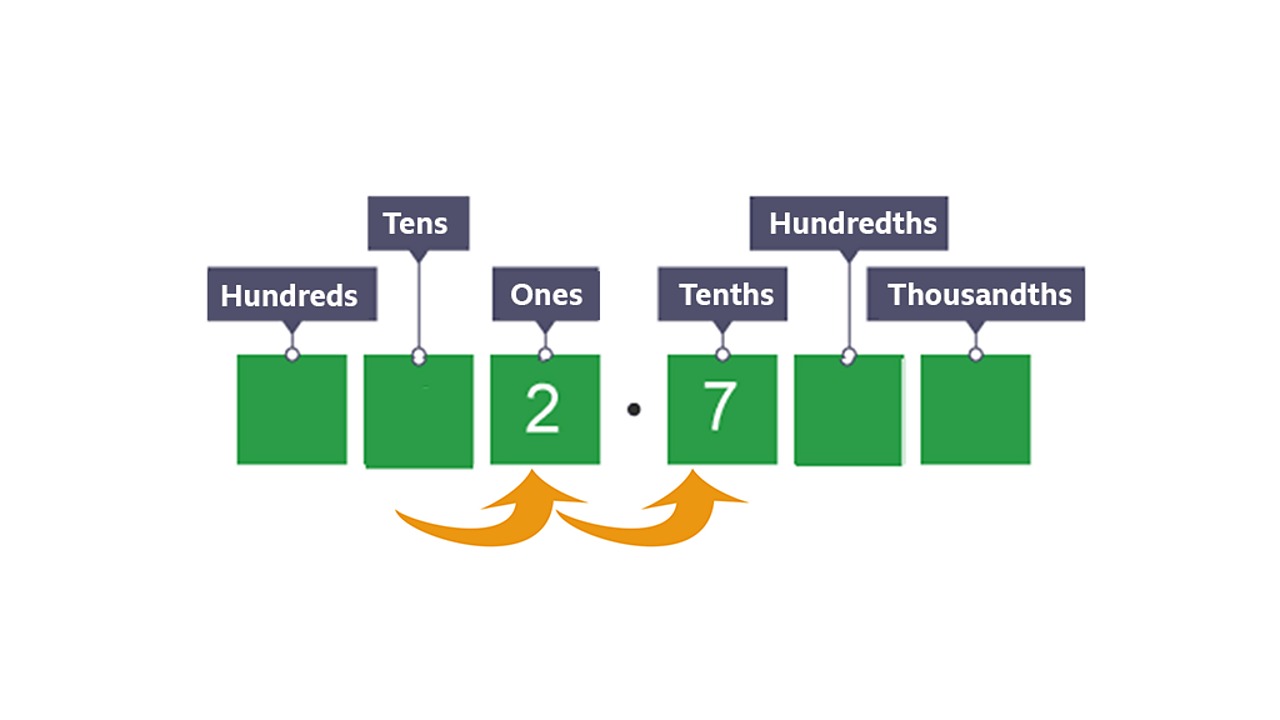To divide by 10, you move the digits one place to the right. So 27 ÷ 10 = 2.7.4 of 4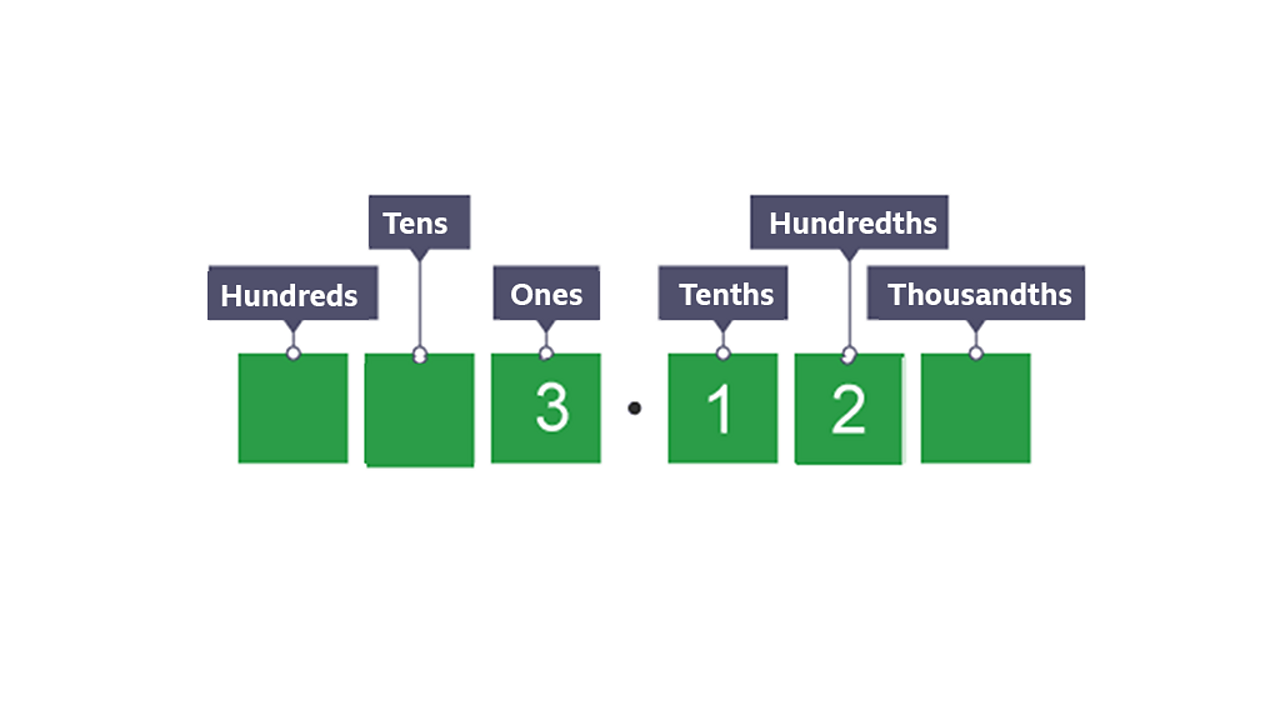Multiplying by 100What is 3.12 × 100?1 of 4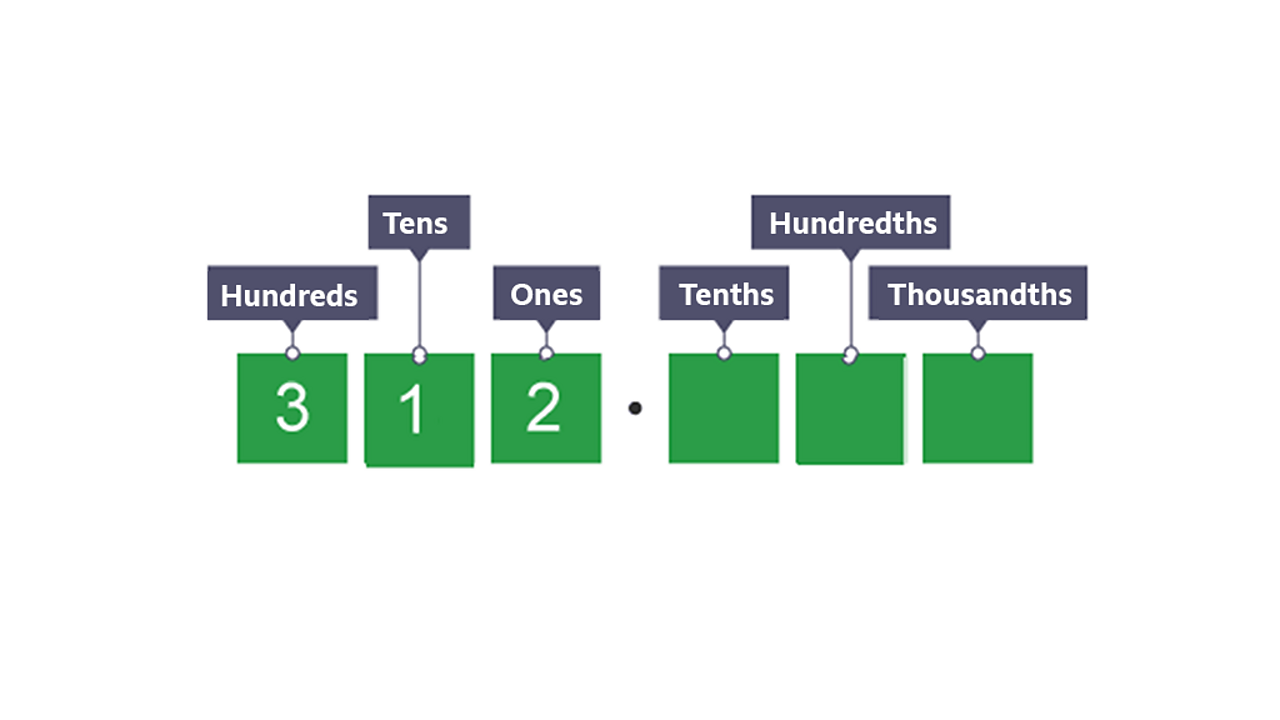To multiply by 100, you move the digits two places to the left. So 3.12 × 100 = 312.2 of 4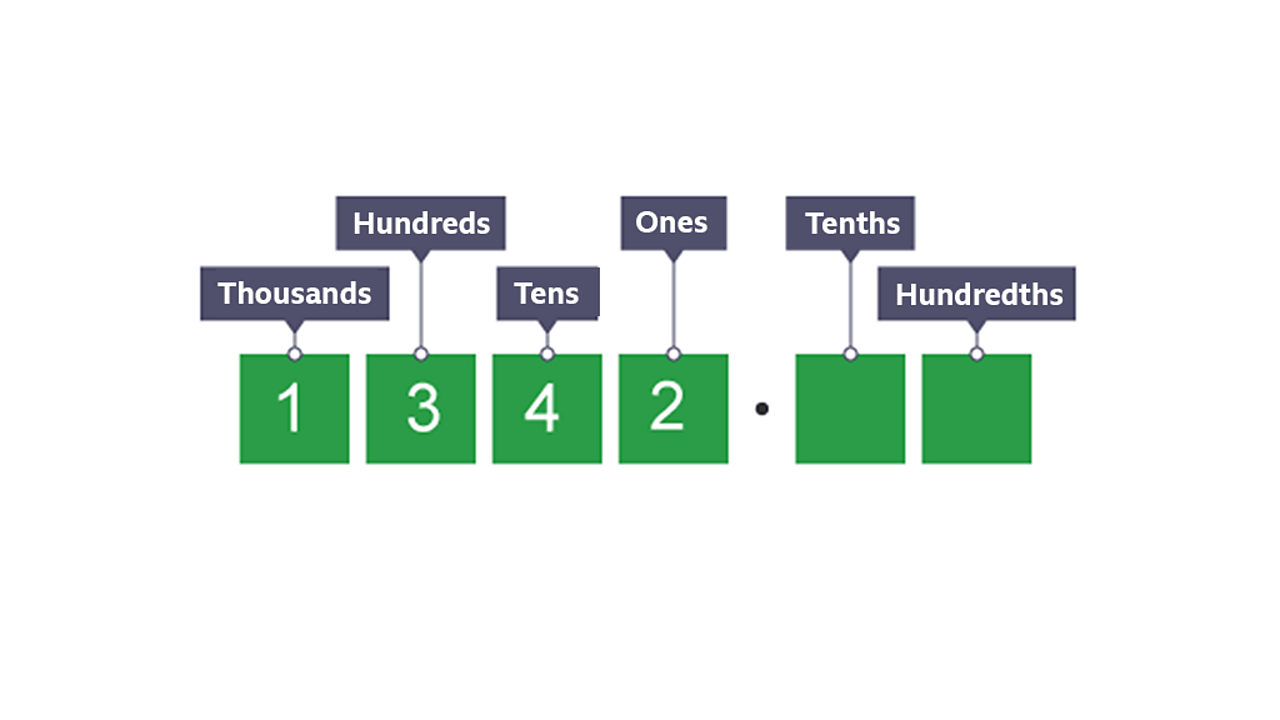Dividing by 100What is 1342 divided by 100?3 of 4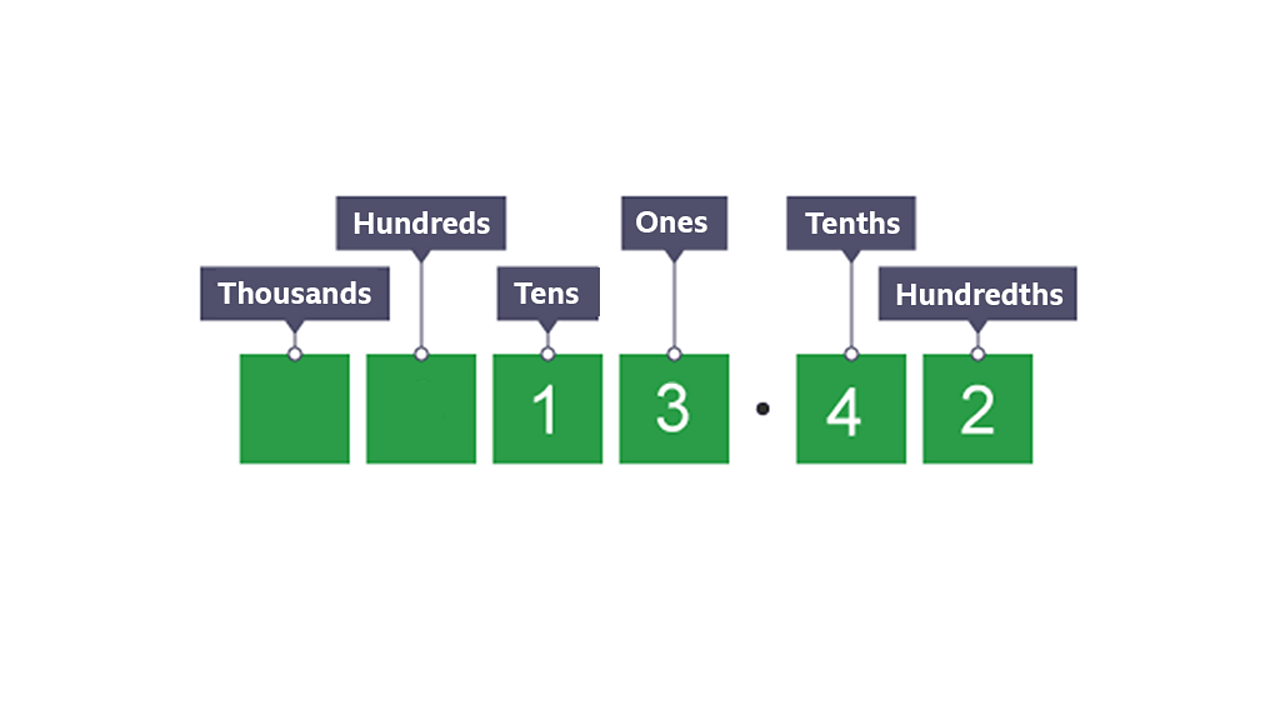To divide by 100, you move the digits two places to the right. So 1342 ÷ 100 = 13.424 of 4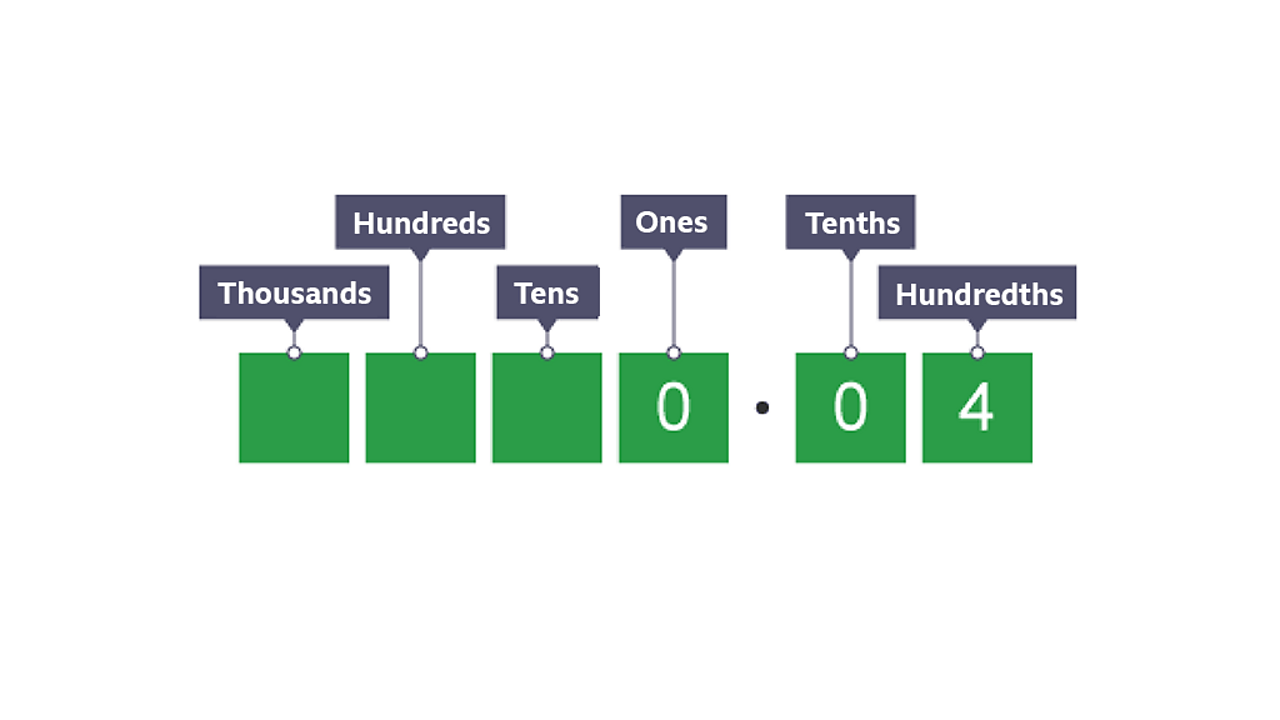Multiplying by 1000What is 0.04 × 1000?1 of 4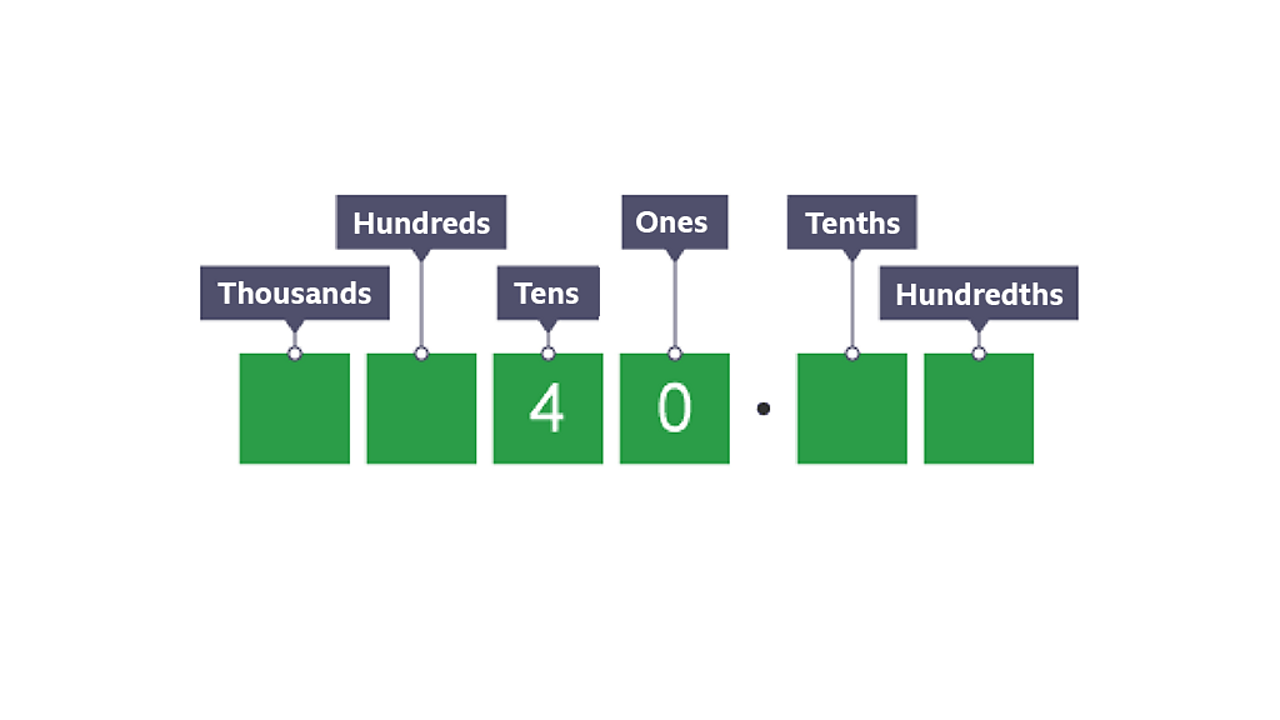To multiply by 1000, you move the digits three place value places to the left. So 0.04 × 1000 = 40.2 of 4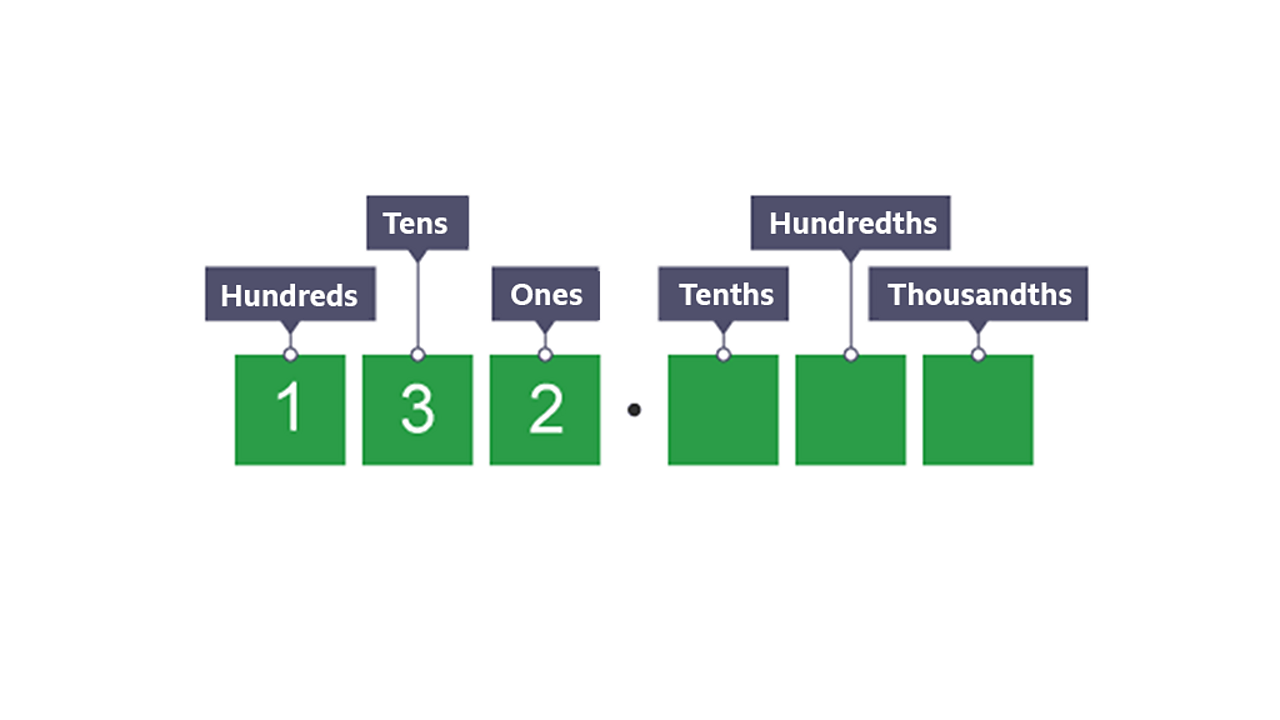Dividing by 1000What is 132 divided by 1000?3 of 4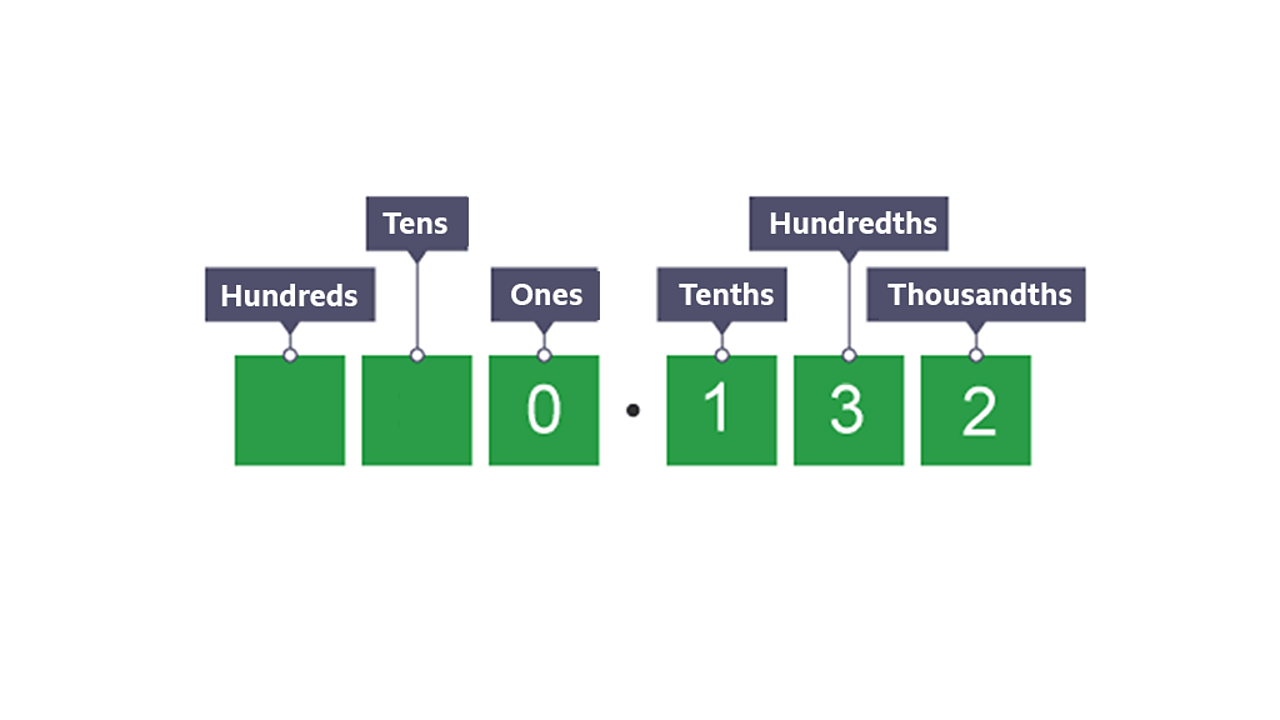To divide by 1000, you move the digits three places to the right. So 132 ÷ 1000 = 0.132. Now the digits are all past the decimal point, we have to add in a zero into the ones column.4 of 4

# Practise

## Activity 1

Multiplying and dividing by 10

Use what you have learnt about place value to multiply and divide these numbers by 10.

## Activity 2

Multiplying and dividing by 10 and 100

Now complete the activities in this worksheet from Collins to multiply and divide the numbers by 10 and 100.